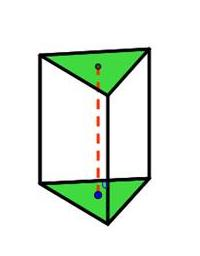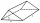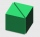# Triangular prism

The base perpendicular triangular prism is a right triangle whose hypotenuse measures 5 cm and one cathetus 2 cm. Height of the prism is equal to 7/9 of the perimeter of the base. Calculate the surface area of prism.

S =  113.5 cm2

### Step-by-step explanation:Did you find an error or inaccuracy? Feel free to write us. Thank you!Tips to related online calculators

#### You need to know the following knowledge to solve this word math problem:

We encourage you to watch this tutorial video on this math problem:

## Related math problems and questions:

• Triangular prismThe base of the perpendicular triangular prism is a rectangular triangle with a hypotenuse of 10 cm and one leg of 8 cm. The prism height is 75% of the perimeter of the base. Calculate the volume and surface of the prism.
• Triangular prismCalculate a triangular prism if it has a rectangular triangle base with a = 4cm and hypotenuse c = 50mm and height of the prism is 0.12 dm.
• Vertical prismThe base of the vertical prism is a right triangle with leg a = 5 cm and a hypotenuse c = 13 cm. The height of the prism is equal to the circumference of the base. Calculate the surface area and volume of the prism
• Triangular prismThe base of the perpendicular triangular prism is a right triangle with a leg length of 5 cm. The content area of the largest sidewall of its surface is 130 cm², and the height of the body is 10 cm. Calculate its volume.
• Triangular prism - regularThe regular triangular prism is 7 cm high. Its base is an equilateral triangle whose height is 3 cm. Calculate the surface and volume of this prism.
• Triangular prismCalculate the surface of a regular triangular prism, the edges of the base are 6 cm long and the height of the prism is 15 cm.
• Triangular prismCalculate the volume and surface of the triangular prism ABCDEF with base of a isosceles triangle. Base's height is 16 cm, leg 10 cm, base height vc = 6 cm. The prism height is 9 cm.
• Paper boxCalculate how much we'll pay for a three-side shaped prism box with a triangular base, and if it measures 12cm and 1.6dm, the hypotenuse measures 200mm. The box is 34cm high. We pay 0,13 € per square meter of paper.
• PrismThe base of a perpendicular triangular prism is a right triangle with legs 4.5 cm and 6 cm long. What is the surface of the prism, if its volume is 54 cubic centimeters?
• 3s prismIt is given a regular perpendicular triangular prism with a height of 19.0 cm and a base edge of 7.1 cm. Calculate the volume of the prism.
• Triangular prismCalculate the surface area and volume of a triangular prism, base right triangle if a = 3 cm, b = 4 cm, c = 5 cm, and height of prism h=12 cm.
• Triangular prismCalculate the volume and surface area of a triangular prism if it is given: a = 6.8 dm. ..Va = 4 dm. (base edge length and base triangle height length) ... ... .v = 23 dm (body height)
• Right triangular prismWe have a cuboid with a base and dimensions of 12 cm and 5 cm and a height of 4 cm. The tablecloth is cut into two identical triangular prisms with right triangular bases. We painted the surface of the created prisms with color. Calculate the surface area
• Triangular prismThe triangular prism has a base in the shape of a right triangle, the legs of which is 9 cm and 40 cm long. The height of the prism is 20 cm. What is its volume cm3? And the surface cm2?
• Regular triangular prismCalculate the surface area of body of regular triangular prism, when the length of its base edge is 6.5 cm and height 0.2 m.
• Triangular prismCalculate the surface of a triangular prism with the base of an equilateral triangle with a side length of 7.5 cm and a corresponding height of 6.5 cm. Prism height is 15cm.
• Base of prismThe base of the perpendicular prism is a rectangular triangle whose legs length are at a 3: 4 ratio. The height of the prism is 2cm smaller than the larger base leg. Determine the volume of the prism if its surface is 468 cm2.